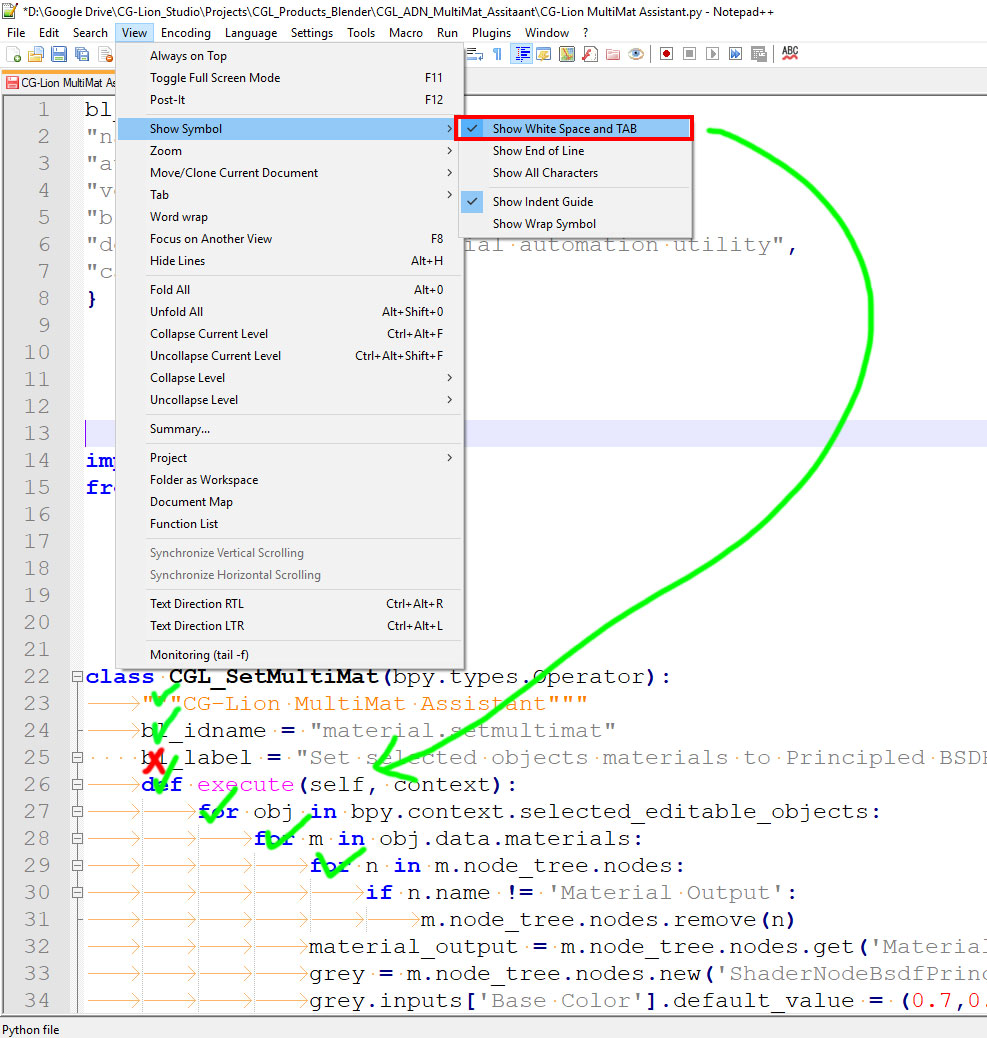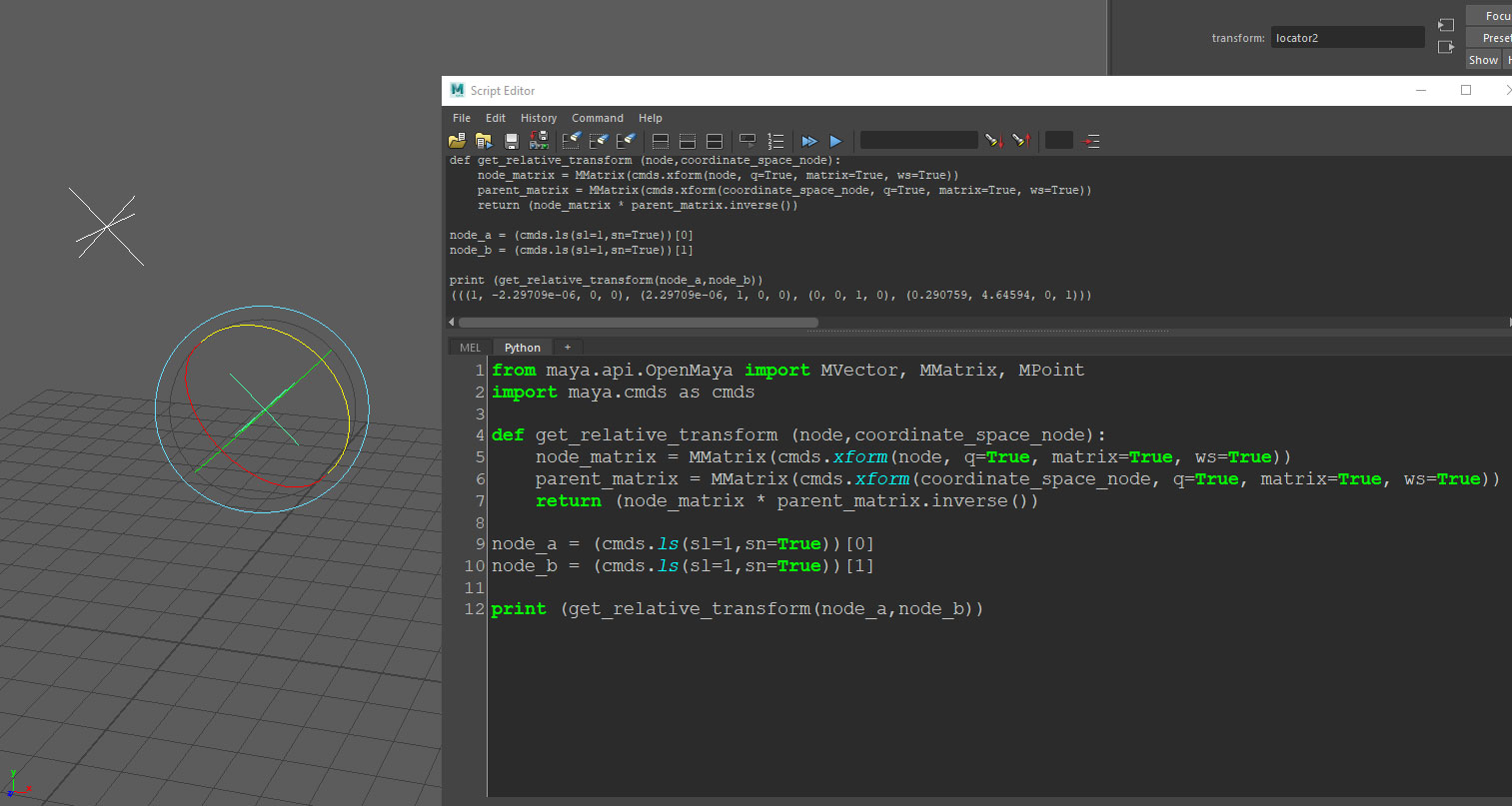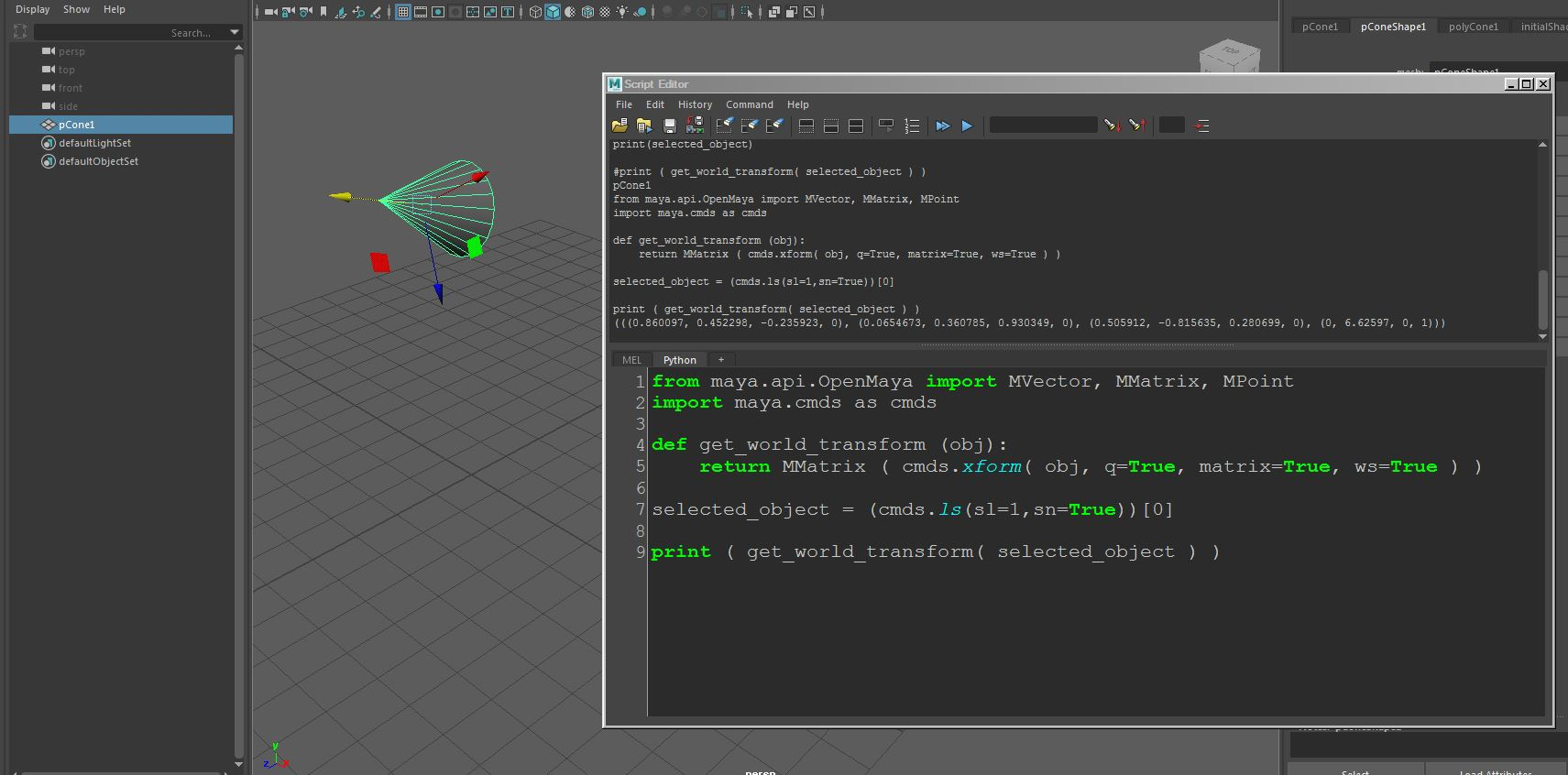## Notepad++ – Show White Space and TAB

Software:

A lot of Python debugging frustration can be avoided by activating the ‘Show White Space and TAB’ option from the View > Show Symbol menu.

This view option displays yellow dots for White Spaces and yellow arrows for TAB indents in the script, and can save precious time locating places where the indentation is wrong.## Maya Python scripting – Getting an object’s transform matrix relative to another object’s coordinates

Software:
Maya 2018

How to get an object transformation matrix relative to another object’s coordinates:
* The following script requires selecting 2 objects, the function will return the transform matrix of the first object relative to the transform matrix of the second.

```from maya.api.OpenMaya import MVector, MMatrix, MPoint
import maya.cmds as cmds

def get_relative_transform (node,coordinate_space_node):
node_matrix = MMatrix(cmds.xform(node, q=True, matrix=True, ws=True))
parent_matrix = MMatrix(cmds.xform(coordinate_space_node, q=True, matrix=True, ws=True))
return (node_matrix * parent_matrix.inverse())

node_a = (cmds.ls(sl=1,sn=True))
node_b = (cmds.ls(sl=1,sn=True))

print (get_relative_transform(node_a,node_b))```## Maya Python scripting – Getting an Object’s world-space transform matrix

Software:
Maya 2018

How to get an object transformation matrix in world space coordinates:

```from maya.api.OpenMaya import MVector, MMatrix, MPoint
import maya.cmds as cmds

def get_world_transform (obj):
return MMatrix ( cmds.xform( obj, q=True, matrix=True, ws=True ) )

selected_object = (cmds.ls(sl=1,sn=True))

print ( get_world_transform( selected_object ) )```